Drug sensitivity and resistance are quantified using IC50 or Emax values, but these metrics suffer from a fundamental flaw when applied to growing cells: they are highly sensitive to the number of divisions that take place over the course of a response assay (see the exploration tool below). Division rate varies across cell lines and experimental conditions. The dependency of IC50 and Emax on division rate creates artefactual correlations between genotype and drug sensitivity while obscuring important biological insights and interfering with biomarker discovery. To address this, we derived alternative drug response metrics that are insensitive to division number. These metrics are based on estimating growth rate inhibition (GR) in the presence of a drug (relative to an untreated control) using endpoint or time-course assays. The latter provides a direct measure of phenomena such as adaptive drug resistance. Theory and experiments published in Hafner et al. (2016) show that GR50 and GRmax are superior to IC50 and Emax for assessing the effects of drugs on dividing cells. Moreover, adopting GR metrics requires only modest changes in experimental protocols. GR metrics should improve the use of drugs to identify response biomarkers, study mechanisms of cell signaling and growth and identify drugs effective on specific patient-derived tumor cells. Through this website we provide an online tool to calculate GR metrics from user-provided data. Scripts are also available on GitHub.Illustration of the dependency of traditional metrics on division time. Simulated data showing relative cell count (green lines) and GR metrics (purple lines) for a cytostatic drug assayed over 3 days across cell lines with different division times. The darker the line, the longer the division time of the cell line (given in days; see key above). IC50 and GR50 are projected onto the x-axis; Emax and GRmax are projected onto the y-axis. Note how the IC curves suggest a more resistant phenotype for slow-growing cell lines whereas the GR curves for all lines overlap, indicating that the GR metrics are independent of the division time. An interactive version of this plot can be found in the Exploration tool tab of this section.

On this website you will find:

• a discussion of traditional metrics based on relative cell count
• a discussion of the new metrics based on normalized growth rate inhibition (GR)
• instructions for calculating GR metrics using our online calculator
• interactive tools for browsing LINCS dose-response datasets that were evaluated using GR metrics

Additional information about measuring dose-response curves and calculating GR metrics can be found in the slides from the: ICSB 2017 Workshop on Drug Response Measurement and Analysis. Applications of GR metrics are further discussed in Hafner et al. (2016) and Hafner et al. (2107). Please cite these paper for all applications of GR metrics.

### Funding Sources

The GR metrics model and the associated computational scripts were developed by members of the Sorger lab at the Harvard Medical School (HMS) LINCS Center, which is funded by NIH grant U54 HL127365. The online GR tools were developed by the LINCS-BD2K Data Coordination and Integration Center, which is funded by NIH grant U54HL127624, in collaboration with the HMS LINCS Center.

### References and resources

1. Hafner M*, Niepel M*, Chung M, Sorger PK. (2016) Growth rate inhibition metrics correct for confounders in measuring sensitivity to cancer drugs. Nat Methods, vol. 13, 521–7. doi: 10.1038/nmeth.3853.
2. Hafner M, Niepel M, Sorger PK. (2017) Alternative drug sensitivity metrics improve preclinical cancer pharmacogenomics. Nat Biotech, vol. 35(6), 500-2. doi: 10.1038/nbt.3882.
3. Clark N*, Hafner M*, Kouril M, Williams EH, Muhlich J, Pilarczyk M, Niepel M, Sorger PK, Medvedovic M. (2017) GRcalculator: an online tool for calculating and mining dose-response data. BMC Cancer, in press.
4. For offline computation, analysis, and visualization, see the Bioconductor R package GRmetrics.
5. ICSB 2017 Workshop on Drug Response Measurement and Analysis
*These authors contributed equally to this work.

Commonly, relative cell count at the end of treatment is used to assess drug response. Across a range of concentrations, the measured cell count or a surrogate of it (e.g. measurement of ATP using CellTiter-Glo®) is normalized to the cell count of DMSO-treated controls grown on the same plate under the same conditions. For each condition (combination of cell line, drug, and drug concentration), we define the relative cell count as x(c)/xctrl, where x(c) is the count in the presence of drug and xctrl is the 50%-trimmed mean of the count for control cells. Based on a sigmoidal curve fitted to the relative cell count across different concentrations, one can define:

• IC50 (50% relative cell count), which is the most commonly used metric but one that can only be defined if the relative cell count decreases during the assay to below 50% of the starting value;
• EC50 (half-point of the sigmoidal curve);
• Einf (the asymptotic efficacy), which differs from Emax (the maximal measured efficacy); and
• AUC (area under, or over, the curve), which captures both IC50 and Emax to some extent and is more robust to experimental noise than other metrics.

More details on dose-response assays and traditional metrics can be found in Sebaugh (2010).Examples of dose-response curves based on relative cell count. In the first example (left), a strong drug response results in an Einf close to 0 and a well-defined IC50. In the second (right), a partial response results in an Einf above 0.5 and an undefined IC50.

As an alternative to traditional metrics, we propose to use GR metrics, which are based on inhibition of the growth rate over the course of the assay and are independent of the division rate of the assayed cell lines.

Growth can be estimated at different concentrations based on the cell count at the time of treatment (x0), the cell count in the untreated control (xctrl), and the cell count after treatment at concentration c (x(c)):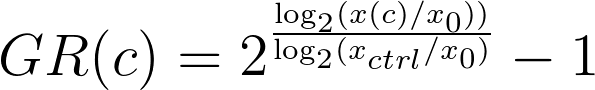This equation assumes exponential growth and constant growth inhibition by the drug. Many phenomena such as drug efflux, homeostasis and adaptation can result in non-exponential growth. In such cases, time course assays and time-dependent GR values are better suited to evaluating drug response. Given measurements of cell count at different time points, time-dependent GR values are defined as: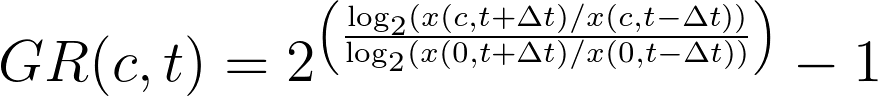GR(c) values (or time-dependent GR(c,t) values at a given time t) across a range of concentrations are fitted to a sigmoidal curve: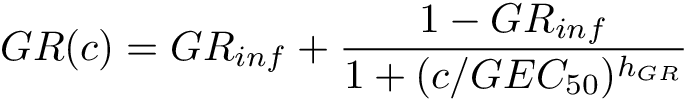where the fitted parameters are:

• GRinf: the effect of the drug at infinite concentration (GRinf = GR(c→)). GRinf lies between -1 and 1. Negative values correspond to cytotoxic responses (i.e., induction of cell death), and a value of 0 corresponds to a fully cytostatic response.
• hGR: the Hill coefficient of the fitted curve, which reflects how steep the dose-response curve is. In practice, we typically constrain hGR to a value between 0.1 and 5.
• GEC50: the concentration at half-maximal effect. To avoid artefacts in curve fitting we constrain GEC50 to be within two orders of magnitude higher and lower than the experimentally tested concentration range. (In practice, this is usually about 10-7 to 103 M.) If the fit of the curve is not significantly better than that of a flat curve (i.e., GR(c)GRinf) based on an F-test with cutoff of p = 0.05, the response is considered flat, and the parameter GEC50 is set to 0.

If time-dependent GR(c,t) values are used, GR metrics can be estimated at a different time points to study how sensitivity evolves over time.

### Inferred drug response metrics

The GR50 value is the concentration of drug at which GR(c = GR50) = 0.5. If the value for GRinf is above 0.5, the GR50 value is not defined and is therefore set to +. By extension, other thresholds can be defined in a similar manner. For example, GR100 corresponds to the concentration at which a drug is fully cytostatic: GR(c = GR100) = 0.

GRmax is the maximum effect of the drug at the highest tested concentration. It lies between -1 and 1. A value of 0 corresponds to a fully cytostatic response, and negative values correspond to a cytotoxic response. GRmax can be estimated from the fitted curve or obtained directly from experimental data. (We often do the later.)

For time course data, all metrics are evaluated at each time point individually.

### Area under the curve and over the curve (GRAOC)

Another common metric for quantifying dose response is the area under the response curve (AUC), which is based on integrating the dose-response curve over the range of tested concentrations. In the case of GR curves, which can have negative values, it is more intuitive to use the area over the curve: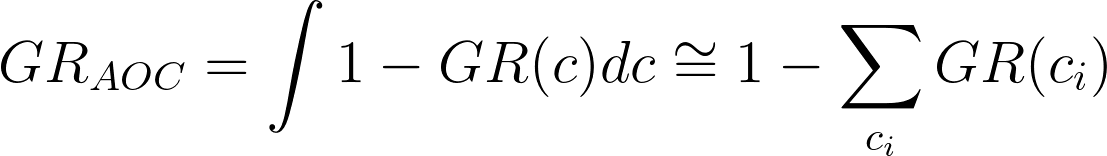where GR(ci) are measured GR values at discrete concentrations ci. GRAOC has the benefit that, in the case of no response, it has a value of 0. It is important to note that GRAOC values (like conventional AUC) can only be used to compare responses evaluated across the same drug concentration range.

The GRAOC value captures variation in potency and efficacy at the same time. The calculation of GRAOC at discrete (experimentally determined) concentrations has the advantage that it does not require curve fitting and is therefore free of fitting artifacts. This is especially useful for assays where fewer than five concentrations are measured and curve fitting is unreliable. GRAOC values are also more robust to experimental noise than metrics derived from curve fitting; e.g. GRmax is particularly sensitive to outlier values when directly obtained from data.

We used computer simulation (see below) to model drug response by three idealized cell lines having identical sensitivity to a partial, or complete cytostatic drug (i.e., a drug that arrests but does not kill cells), or a cytotoxic drug (i.e., a drug that kills cells) and different division times (Td). For example, the lower quartile, median, and upper quartile of the division times for breast cancer cell lines has been reported as 1.8, 2.4, or 3.9 days, respectively (Heiser et al. (2012)). These values are similar to those of NCI-60 cells. In a slow-dividing cell line (Td > 3.9 d), the total number of cells does not double in a typical three-day assay; thus Emax ≥ 0.5 and IC50 are undefined. In the case of the two faster-growing cell lines, IC50 and Emax values fall as division rate increases simply because cell number (or CTG value) is normalized to a drug-naïve control in which cell number increases as division time falls.

 Cell division time (Td) 1.3 Potency of the drug (SC50) 1.2 Maximal effect of the drug 0.65 on cell division (SM) Hill slope (h) 1.6

To simulate the effect of division time on GR and conventional drug-response metrics under different assumptions about the degree of cytostasis or cell killing, we developed a theoretical model of drug response. To the first approximation, cell growth can be considered exponential, with drugs either decreasing the division rate or killing cells in a cell cycle-dependent manner: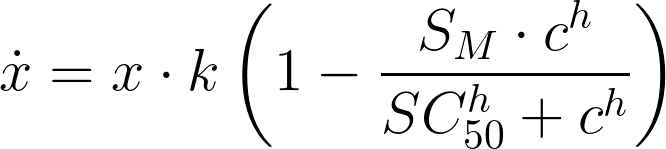where x is the cell count, k is the untreated growth rate (per day), c is the drug concentration, SM is the maximal inhibitory effect, SC50 is the concentration at half-maximal effect of drug, and h is the Hill coefficient. The growth rate k corresponds to the division rate k0 as k = ln(2)⋅k0 = ln(2)/Td, where Td is the division time. SM can be larger than 1 to account for drugs inducing cell death at a specific phase of the cell cycle.

Integrating these equations for an assay of t days yields the cell count at concentration c: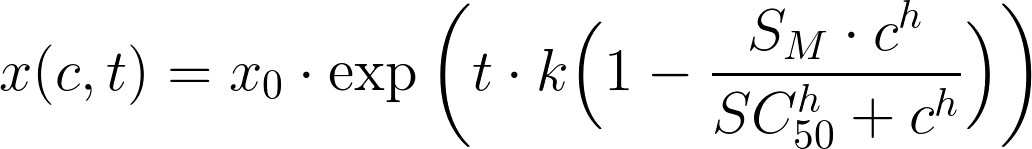where x0 = x(t = 0) is the cell number at the time of treatment. Thus, the relative cell count is: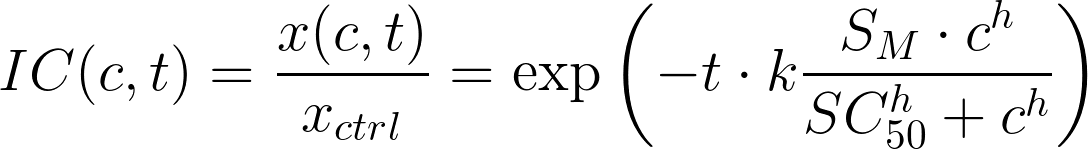where xctrl ≡ x(0), and the normalized growth rate inhibition (GR value) is: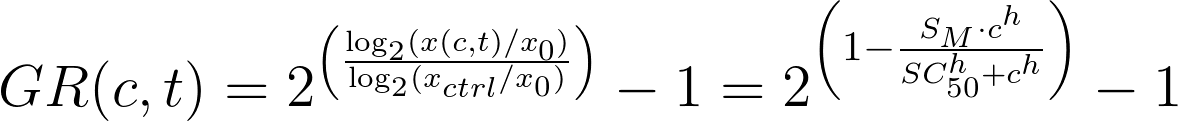This equation for GR(c) is independent of the length of the assay t and the untreated growth rate k, and, thus, the metrics GR50, GRmax, GRAOC, and hGR are also independent of t and k.

Through the accompanying webpages we provide online tools that allow users to upload their own endpoint data, calculate the corresponding GR values and GR metrics, and compare their results across the variables in the assay, such as cell line, agent (drug), time and replicate. Through the above Online GR Calculator webpage, we provide online tools that allow users to upload their own endpoint data, calculate the corresponding GR values and GR metrics, and compare their results across the variables in the assay, such as cell line, agent (drug), time and replicate. Python, R and MATLAB code for calculating GR value and GR metrics is also available in the gr_metrics repository on GitHub. Our R code is also available in the Bioconductor package GRmetrics.

## Input data

The GRcalculator takes in either comma-separated (.csv) or tab-separated (.tsv) files with specified column names. See the step-by-step example. For more explicit instructions, go to the GRcalculator and click "Import Data File" and select a "GR Calculation Method" and "Input File Format" to see the exact columns needed for a particular input style.

## Calculated metrics

The following GR metrics are calculated:

• GR50: The concentration at which the effect reaches a GR value of 0.5 based on interpolation of the fitted curve.
• GRmax: The effect at the highest tested concentration.
• GRinf = GR(c → ∞): The effect at infinite concentration based on extrapolation of the fitted curve, which reflects asymptotic drug efficacy. Note that GRinf can differ from GRmax if the measured dose-response does not reach its plateau value.
• GEC50, the drug concentration at half-maximal effect, which reflects the potency of the drug.
• hGR: Hill coefficient of the sigmoidal curve, which reflects how steep the dose-response curve is.
• GRAOC: The area over the dose-response curve, which is the integral of 1–GR(c) over the range of concentrations tested.

In addition, the online calculator reports the r-squared of the fit and evaluates the significance of the sigmoidal fit based on an F-test. Further information about these calculations is available through our GR tutorial. Traditional metrics based on relative cell count (e.g. IC50, Emax, etc.) are computed as well for comparison.

The results of analysis of existing LINCS dose-response datasets using the GR model are available in the LINCS GR Data Browser webpage.

## Analysis and visualization tools

The following tabs allow users to analyze and visualize their data and the calculated GR metrics:

• Data Tables displays the uploaded data and calculated GR values as well as the inferred GR metrics. Data can be downloaded in .csv or .tsv format.
• Dose-Response by Condition plots the data points and fitted dose-response curves for each condition. If no specific conditions are selected, all data will be overlaid on a single plot. To display a subset of the data, select specific conditions to display (e.g. a specific cell line or drug). Mousing over the plot displays the GR values and the corresponding concentration. Plots can be downloaded in a variety of image formats.
• Dose-Response Grid shows the fitted dose-response curves side by side and grouped according to the selected variables. ‘Selector variable’ and ‘grid variable’ define which plots are displayed. ‘Selector variable’ also defines the variable displayed in the selector box to the left of the plots.
• GR Metric Comparison shows a scatter plot of the a given GR metric across different conditions. Users can select which metric to plot relative to which experimental variable. Mousing over the plot displays the plotted GR metrics. Plots can be downloaded in a variety of image formats.